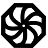# Programming-Idioms# 169
Implementation
VB

Be concise.

Be useful.

All contributions dictatorially edited by webmasters to match personal tastes.

Please try to avoid dependencies to third-party libraries and frameworks.

Implementation edit is for fixing errors and enhancing with metadata.

Instead of changing the code of the snippet, consider creating another VB implementation.

Other implementations
`int n = s.length();`
`const n = s.length;`
`import "unicode/utf8"`
`n := utf8.RuneCountInString(s)`
`n = s.length`
`uses LazUtf8;`
`n := length(s);`
`int n = s.length;`
`n = s.size`
`uses LazUtf8;`
`n := Utf8Length(s);`
`size_t n = s.length;`
`std.range;`
`size_t n = s.walkLength;`
`import std.uni, std.range;`
`size_t n = s.byGrapheme.walkLength;`
`n = len(s)`
`n = length s`
`uses sysutils;`
`n := s.length;`
`n = String.length s`
`local n = utf8.len(s)`
`let n = s.chars().count();`
`my \$n = length( \$s );`
`\$n = mb_strlen(\$s, 'UTF-8');`
`int n = s.Length;`
`val n = s.length()`
`(setf n (length s))`
`(length s)`
`(def n (.codePointCount s 0 (count s)))`
`n = len(s)`
`val n = s.length`
`N = string:length(S)`
```IDENTIFICATION DIVISION.
PROGRAM-ID. length.
PROCEDURE DIVISION.
MOVE FUNCTION LENGTH(s) TO n
STOP RUN.```
```s : String := "Hello World";
n : Integer := s'Length;```